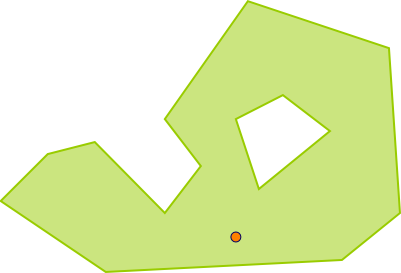#Boost C++ Libraries

...one of the most highly regarded and expertly designed C++ library projects in the world.

This is the documentation for an old version of Boost. Click here to view this page for the latest version.
##### within

Checks if the first geometry is completely inside the second geometry.

###### Description

The free function within checks if the first geometry is completely inside the second geometry.

###### Synopsis

```template<typename Geometry1, typename Geometry2>
bool within(Geometry1 const & geometry1, Geometry2 const & geometry2)```

###### Parameters

Type

Concept

Name

Description

Geometry1 const &

Any type fulfilling a Geometry Concept

geometry1

geometry which might be within the second geometry

Geometry2 const &

Any type fulfilling a Geometry Concept

geometry2

geometry which might contain the first geometry

###### Returns

true if geometry1 is completely contained within geometry2, else false

###### Header

Either

`#include <boost/geometry/geometry.hpp>`

Or

`#include <boost/geometry/algorithms/within.hpp>`

###### Supported geometries

Box

Ring

Polygon

MultiPolygon

Point###### Notes

If a point is located exactly on the border of a geometry, the result depends on the strategy. The default strategy (Winding (coordinate system agnostic)) returns false in that case.

If a polygon has a reverse oriented (e.g. counterclockwise for a clockwise typed polygon), the result also depends on the strategy. The default strategy returns still true if a point is completely within the reversed polygon. There is a specific strategy which returns false in this case.

Linear

###### Example

Shows how to detect if a point is inside a polygon, or not

```#include <iostream>
#include <list>

#include <boost/geometry.hpp>
#include <boost/geometry/geometries/point_xy.hpp>
#include <boost/geometry/geometries/polygon.hpp>

#include <boost/geometry/domains/gis/io/wkt/wkt.hpp>

int main()
{
typedef boost::geometry::model::d2::point_xy<double> point_type;
typedef boost::geometry::model::polygon<point_type> polygon_type;

polygon_type poly;
boost::geometry::read_wkt(
"POLYGON((2 1.3,2.4 1.7,2.8 1.8,3.4 1.2,3.7 1.6,3.4 2,4.1 3,5.3 2.6,5.4 1.2,4.9 0.8,2.9 0.7,2 1.3)"
"(4.0 2.0, 4.2 1.4, 4.8 1.9, 4.4 2.2, 4.0 2.0))", poly);

point_type p(4, 1);

std::cout << "within: " << (boost::geometry::within(p, poly) ? "yes" : "no") << std::endl;

return 0;
}

```

Output:

```within: yes```
 Copyright © 2011 Barend Gehrels, Bruno Lalande, Mateusz Loskot Distributed under the Boost Software License, Version 1.0. (See accompanying file LICENSE_1_0.txt or copy at http://www.boost.org/LICENSE_1_0.txt)